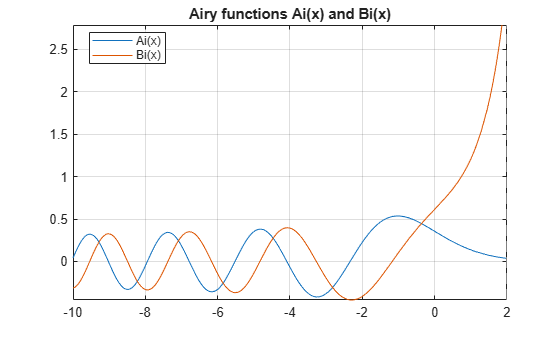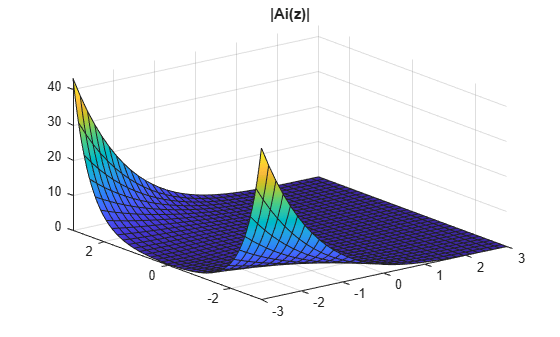# airy

## Syntax

``airy(x)``
``airy(0,x)``
``airy(1,x)``
``airy(2,x)``
``airy(3,x)``
``airy(n,x)``
``airy(___,1)``

## Description

example

````airy(x)` returns the Airy function of the first kind, Ai(x), for each element of `x`.```
````airy(0,x)` is the same as `airy(x)`.```

example

````airy(1,x)` returns the derivative of Ai(x).```

example

````airy(2,x)` returns the Airy function of the second kind, Bi(x).```

example

````airy(3,x)` returns the derivative of Bi(x).```
````airy(n,x)` uses the values in vector `n` to return the corresponding Airy functions of elements of vector `x`. Both `n` and `x` must have the same size.```
````airy(___,1)` returns the Scaled Airy Functions following the syntax for the MATLAB® `airy` function.```

## Examples

### Find the Airy Function of the First Kind

Find the Airy function of the first kind, Ai(x), for numeric or symbolic inputs using `airy`. Approximate exact symbolic outputs using `vpa`.

Find the Airy function of the first kind, Ai(x), at `1.5`. Because the input is double and not symbolic, you get a double result.

`airy(1.5)`
```ans = 0.0717```

Find the Airy function of the values of vector `v` symbolically, by converting `v` to symbolic form using `sym`. Because the input is symbolic, `airy` returns exact symbolic results. The exact symbolic results for most symbolic inputs are unresolved function calls.

```v = sym([-1 0 25.1 1+1i]); vAiry = airy(v)```
```vAiry = [ airy(0, -1), 3^(1/3)/(3*gamma(2/3)), airy(0, 251/10), airy(0, 1 + 1i)]```

Numerically approximate the exact symbolic result using `vpa`.

`vpa(vAiry)`
```ans = [ 0.53556088329235211879951656563887, 0.35502805388781723926006318600418,... 4.9152763177499054787371976959487e-38,... 0.060458308371838149196532978116646 - 0.15188956587718140235494791259223i] ```

Find the Airy function, Ai(x), of the symbolic input `x^2`. For symbolic expressions, `airy` returns an unresolved call.

```syms x airy(x^2)```
```ans = airy(0, x^2)```

### Find the Airy Function of the Second Kind

Find the Airy function of the second kind, Bi(x), of the symbolic input `[-3 4 1+1i x^2]` by specifying the first argument as `2`. Because the input is symbolic, `airy` returns exact symbolic results. The exact symbolic results for most symbolic inputs are unresolved function calls.

```v = sym([-3 4 1+1i x^2]); vAiry = airy(2, v)```
```vAiry = [ airy(2, -3), airy(2, 4), airy(2, 1 + 1i), airy(2, x^2)]```

Use the syntax `airy(2,x)` like `airy(x)`, as described in the example Find the Airy Function of the First Kind.

### Plot Airy Functions

Plot the Airy Functions, $Ai\left(x\right)$ and $Bi\left(x\right)$, over the interval `[-10 2]` using `fplot`.

```syms x fplot(airy(x), [-10 2]) hold on fplot(airy(2,x), [-10 2]) legend('Ai(x)','Bi(x)','Location','Best') title('Airy functions Ai(x) and Bi(x)') grid on```Plot the absolute value of $Ai\left(z\right)$ over the complex plane.

```syms y z = x + 1i*y; figure(2) fsurf(abs(airy(z))) title('|Ai(z)|') a = gca; a.ZLim = [0 10]; caxis([0 10])```### Find Derivatives of Airy Functions

Find the derivative of the Airy function of the first kind, Ai′(x), at `0` by specifying the first argument of `airy` as `1`. Then, numerically approximate the derivative using `vpa`.

```dAi = airy(1, sym(0)) dAi_vpa = vpa(dAi)```
```dAi = -(3^(1/6)*gamma(2/3))/(2*pi) dAi_vpa = -0.2588194037928067984051835601892```

Find the derivative of the Airy function of the second kind, Bi′(x), at `x` by specifying the first argument as `3`. Then, find the derivative at x = 5 by substituting for `x` using `subs` and calling `vpa`.

```syms x dBi = airy(3, x) dBi_vpa = vpa(subs(dBi, x, 5))```
```dBi = airy(3, x) dBi_vpa = 1435.8190802179825186717212380046```

### Solve Airy Differential Equation for Airy Functions

Show that the Airy functions Ai(x) and Bi(x) are the solutions of the differential equation

`$\frac{{\partial }^{2}y}{\partial {x}^{2}}-xy=0.$`

```syms y(x) dsolve(diff(y, 2) - x*y == 0)```
```ans = C1*airy(0, x) + C2*airy(2, x)```

### Differentiate Airy Functions

Differentiate expressions containing `airy`.

```syms x y diff(airy(x^2)) diff(diff(airy(3, x^2 + x*y -y^2), x), y)```
```ans = 2*x*airy(1, x^2) ans = airy(2, x^2 + x*y - y^2)*(x^2 + x*y - y^2) +... airy(2, x^2 + x*y - y^2)*(x - 2*y)*(2*x + y) +... airy(3, x^2 + x*y - y^2)*(x - 2*y)*(2*x + y)*(x^2 + x*y - y^2) ```

### Expand Airy Function using Taylor Series

Find the Taylor series expansion of the Airy functions, Ai(x) and Bi(x), using `taylor`.

```aiTaylor = taylor(airy(x)) biTaylor = taylor(airy(2, x))```
```aiTaylor = - (3^(1/6)*gamma(2/3)*x^4)/(24*pi) + (3^(1/3)*x^3)/(18*gamma(2/3))... - (3^(1/6)*gamma(2/3)*x)/(2*pi) + 3^(1/3)/(3*gamma(2/3)) biTaylor = (3^(2/3)*gamma(2/3)*x^4)/(24*pi) + (3^(5/6)*x^3)/(18*gamma(2/3))... + (3^(2/3)*gamma(2/3)*x)/(2*pi) + 3^(5/6)/(3*gamma(2/3))```

### Fourier Transform of Airy Function

Find the Fourier transform of the Airy function Ai(x) using `fourier`.

```syms x aiFourier = fourier(airy(x))```
```aiFourier = exp((w^3*1i)/3)```

### Numeric Roots of Airy Function

Find a root of the Airy function Ai(x) numerically using `vpasolve`.

```syms x vpasolve(airy(x) == 0, x)```
```ans = -226.99630507523600716771890962744```

Find a root in the interval `[-5 -3]`.

`vpasolve(airy(x) == 0, x, [-5 -3])`
```ans = -4.0879494441309706166369887014574```

## Input Arguments

collapse all

Input, specified as a number, vector, matrix, or multidimensional array, or a symbolic number, variable, vector, matrix, multidimensional array, function, or expression.

Type of Airy function, specified as a number, vector, matrix, or multidimensional array, or a symbolic number, variable, vector, matrix, or multidimensional array. The values of the input must be `0`, `1`, `2`, or `3`, which specify the Airy function as follows.

n

Returns

`0` (default)

Airy function, Ai(x), which is the same as `airy(x)`.

`1`

Derivative of Airy function, Ai’(x).

`2`

Airy function of the second kind, Bi(x).

`3`

Derivative of Airy function of the second kind, Bi’(x).

collapse all

### Airy Functions

The Airy functions Ai(x) and Bi(x) are the two linearly independent solutions of the differential equation

`$\frac{{\partial }^{2}y}{\partial {x}^{2}}-xy=0.$`

Ai(x) is called the Airy function of the first kind. Bi(x) is called the Airy function of the second kind.

### Scaled Airy Functions

The Airy function of the first kind, Ai(x), is scaled as

`${e}^{\left(\frac{2}{3}{x}^{\left(3/2\right)}\right)}\text{Ai}\left(x\right).$`

The derivative, Ai’(x), is scaled by the same factor.

The Airy function of the second kind, Bi(x), is scaled as

`${e}^{-|\frac{2}{3}\text{Re}\left({x}^{\left(3/2\right)}\right)|}\text{Bi}\left(x\right).$`

The derivative, Bi’(x), is scaled by the same factor.

## Tips

• When you call `airy` for inputs that are not symbolic objects, you call the MATLAB `airy` function.

• When you call `airy(n, x)`, at least one argument must be a scalar or both arguments must be vectors or matrices of the same size. If one argument is a scalar and the other is a vector or matrix, `airy(n,x)` expands the scalar into a vector or matrix of the same size as the other argument with all elements equal to the scalar.

• `airy` returns special exact values at `0`.

## Version History

Introduced in R2012a